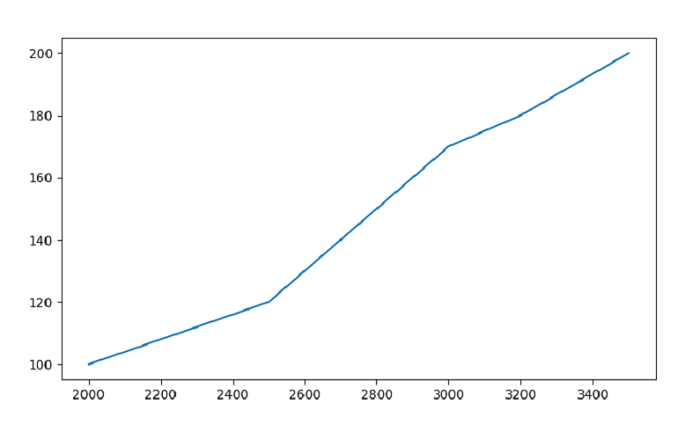# Plot a Line Graph for Pandas Dataframe with Matplotlib?

We will plot a line grapg for Pandas DataFrame using the plot(). At first, import the required libraries −

import pandas as pd
import matplotlib.pyplot as plt

Create a DataFrame −

dataFrame = pd.DataFrame(
{
"Car": ['BMW', 'Lexus', 'Audi', 'Mustang', 'Bentley', 'Jaguar'],"Reg_Price": [2000, 2500, 2800, 3000, 3200, 3500],"Units": [100, 120, 150, 170, 180, 200]
}
)

Plot a line graph with both the columns −

plt.plot(dataFrame["Reg_Price"], dataFrame["Units"])

## Example

Following is the code −

import pandas as pd
import matplotlib.pyplot as plt

# creating a DataFrame with 2 columns
dataFrame = pd.DataFrame(
{
"Car": ['BMW', 'Lexus', 'Audi', 'Mustang', 'Bentley', 'Jaguar'],"Reg_Price": [2000, 2500, 2800, 3000, 3200, 3500],"Units": [100, 120, 150, 170, 180, 200]
}
)

# plot a line graph
plt.plot(dataFrame["Reg_Price"], dataFrame["Units"])
plt.show()

## Output

This will produce the following output −